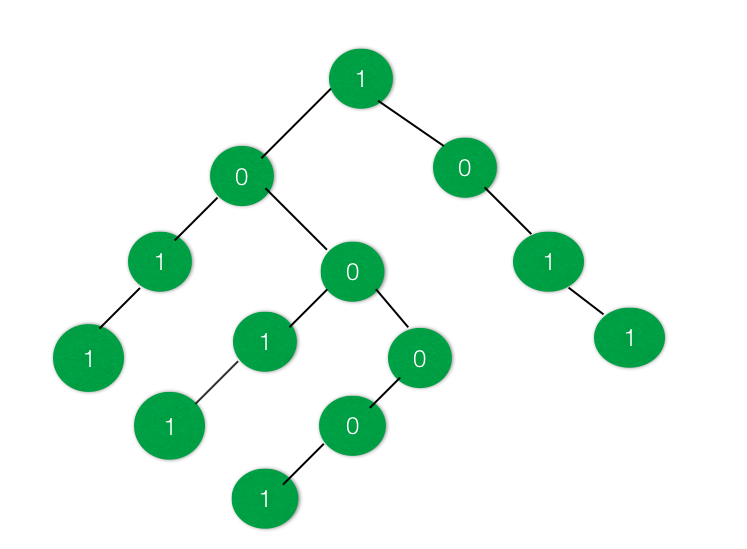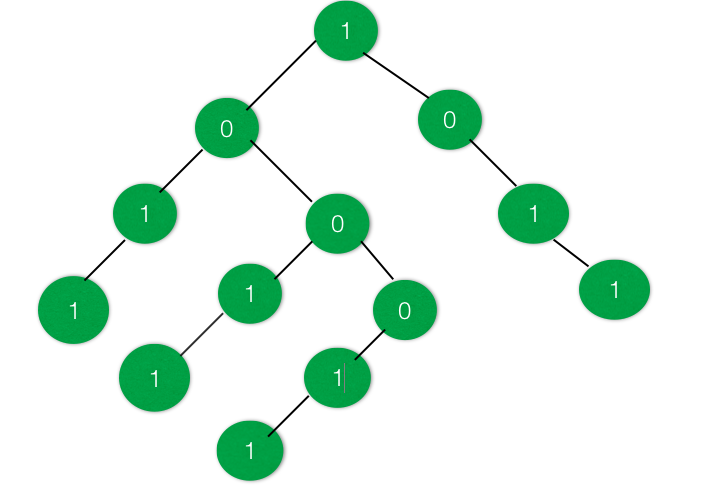Related Articles
Check if the given Binary Tree have a Subtree with equal no of 1’s and 0’s
• Difficulty Level : Hard
• Last Updated : 29 Oct, 2020

Given a Binary Tree having data at nodes as either 0’s or 1’s. The task is to find out whether there exists a subtree having an equal number of 1’s and 0’s.

Examples:

InputOutput : True
There are two subtrees present in the above tree where the number of 1’s is equal to the number of 0’s.

InputOutput : False
There is no such subtree present which has the number of 1’s equal to number of 0’s

Approach: The idea is to change the data 0’s of the tree to -1. So that it becomes very easy to find the subtree having equal number of 0’s and 1’s. After converting all 0’s to -1, create a sum tree. After creating the sum tree, each node will contain the sum of all node lying under it.

Traverse the tree again and find if there is a node having 0 sum, it means that there is a subtree that has the equal number of 1’s and -1’s, i.e. equal number of 1’s and 0’s.

Below is the implementation of the above approach:

## C++

 `// C++ program to check if there exist a` `// subtree with equal number of 1's and 0's`   `#include ` `using` `namespace` `std;`   `// Binary Tree Node` `struct` `node {` `    ``int` `data;` `    ``struct` `node *right, *left;` `};`   `// Utility function to create a new node` `struct` `node* newnode(``int` `key)` `{` `    ``struct` `node* temp = ``new` `node;` `    ``temp->data = key;` `    ``temp->right = NULL;` `    ``temp->left = NULL;`   `    ``return` `temp;` `}`   `// Function to convert all 0's in the` `// tree to -1` `void` `convert(``struct` `node* root)` `{` `    ``if` `(root == NULL) {` `        ``return``;` `    ``}`   `    ``// Move to right subtree` `    ``convert(root->right);`   `    ``// Replace the 0's with -1 in the tree` `    ``if` `(root->data == 0) {` `        ``root->data = -1;` `    ``}`   `    ``// Move to left subtree` `    ``convert(root->left);` `}`   `// Function to convert the tree to a SUM tree` `int` `sum_tree(``struct` `node* root)` `{` `    ``int` `a = 0, b = 0;`   `    ``if` `(root == NULL) {` `        ``return` `0;` `    ``}`   `    ``a = sum_tree(root->left);` `    ``b = sum_tree(root->right);`   `    ``root->data = root->data + a + b;`   `    ``return` `root->data;` `}`   `// Function to check if there exists a subtree` `// with equal no of 1s and 0s` `int` `checkSubtree(``struct` `node* root, ``int` `d)` `{` `    ``if` `(root == NULL) {` `        ``return` `0;` `    ``}`   `    ``// Check if there is a subtree with equal` `    ``// 1s and 0s or not` `    ``if` `(d == 0) {` `        ``d = checkSubtree(root->left, d);` `    ``}`   `    ``if` `(root->data == 0) {` `        ``d = 1;` `        ``return` `d;` `    ``}`   `    ``if` `(d == 0) {` `        ``d = checkSubtree(root->right, d);` `    ``}`   `    ``return` `d;` `}`   `// Driver Code` `int` `main()` `{` `    ``// Create the Binary Tree` `    ``struct` `node* root = newnode(1);` `    ``root->right = newnode(0);` `    ``root->right->right = newnode(1);` `    ``root->right->right->right = newnode(1);` `    ``root->left = newnode(0);` `    ``root->left->left = newnode(1);` `    ``root->left->left->left = newnode(1);` `    ``root->left->right = newnode(0);` `    ``root->left->right->left = newnode(1);` `    ``root->left->right->left->left = newnode(1);` `    ``root->left->right->right = newnode(0);` `    ``root->left->right->right->left = newnode(0);` `    ``root->left->right->right->left->left = newnode(1);`   `    ``// Convert all 0s in tree to -1` `    ``convert(root);`   `    ``// Convert the tree into a SUM tree` `    ``sum_tree(root);`   `    ``// Check if required Subtree exists` `    ``int` `d = 0;` `    ``if` `(checkSubtree(root, d)) {` `        ``cout << ``"True"` `<< endl;` `    ``}` `    ``else` `{` `        ``cout << ``"False"` `<< endl;` `    ``}`   `    ``return` `0;` `}`

## Java

 `// Java program to check if there exist a` `// subtree with equal number of 1's and 0's`   `import` `java.util.*;` `class` `GFG{`   `    ``// Binary Tree Node` `    ``static` `class` `node {` `        ``int` `data;` `        ``node right, left;` `    ``};` `    `  `    ``// Utility function to create a new node` `    ``static` `node newnode(``int` `key)` `    ``{` `        ``node temp = ``new` `node();` `        ``temp.data = key;` `        ``temp.right = ``null``;` `        ``temp.left = ``null``;` `    `  `        ``return` `temp;` `    ``}` `    `  `    ``// Function to convert all 0's in the` `    ``// tree to -1` `    ``static` `void` `convert(node root)` `    ``{` `        ``if` `(root == ``null``) {` `            ``return``;` `        ``}` `    `  `        ``// Move to right subtree` `        ``convert(root.right);` `    `  `        ``// Replace the 0's with -1 in the tree` `        ``if` `(root.data == ``0``) {` `            ``root.data = -``1``;` `        ``}` `    `  `        ``// Move to left subtree` `        ``convert(root.left);` `    ``}` `    `  `    ``// Function to convert the tree to a SUM tree` `    ``static` `int` `sum_tree(node root)` `    ``{` `        ``int` `a = ``0``, b = ``0``;` `    `  `        ``if` `(root == ``null``) {` `            ``return` `0``;` `        ``}` `    `  `        ``a = sum_tree(root.left);` `        ``b = sum_tree(root.right);` `    `  `        ``root.data = root.data + a + b;` `    `  `        ``return` `root.data;` `    ``}` `    `  `    ``// Function to check if there exists a subtree` `    ``// with equal no of 1s and 0s` `    ``static` `int` `checkSubtree(node root, ``int` `d)` `    ``{` `        ``if` `(root == ``null``) {` `            ``return` `0``;` `        ``}` `    `  `        ``// Check if there is a subtree with equal` `        ``// 1s and 0s or not` `        ``if` `(d == ``0``) {` `            ``d = checkSubtree(root.left, d);` `        ``}` `    `  `        ``if` `(root.data == ``0``) {` `            ``d = ``1``;` `            ``return` `d;` `        ``}` `    `  `        ``if` `(d == ``0``) {` `            ``d = checkSubtree(root.right, d);` `        ``}` `    `  `        ``return` `d;` `    ``}` `    `  `    ``// Driver Code` `    ``public` `static` `void` `main(String args[])` `    ``{` `        ``// Create the Binary Tree` `        ``node root = newnode(``1``);` `        ``root.right = newnode(``0``);` `        ``root.right.right = newnode(``1``);` `        ``root.right.right.right = newnode(``1``);` `        ``root.left = newnode(``0``);` `        ``root.left.left = newnode(``1``);` `        ``root.left.left.left = newnode(``1``);` `        ``root.left.right = newnode(``0``);` `        ``root.left.right.left = newnode(``1``);` `        ``root.left.right.left.left = newnode(``1``);` `        ``root.left.right.right = newnode(``0``);` `        ``root.left.right.right.left = newnode(``0``);` `        ``root.left.right.right.left.left = newnode(``1``);` `    `  `        ``// Convert all 0s in tree to -1` `        ``convert(root);` `    `  `        ``// Convert the tree into a SUM tree` `        ``sum_tree(root);` `    `  `        ``// Check if required Subtree exists` `        ``int` `d = ``0``;` `        ``if` `(checkSubtree(root, d)>=``1``) {` `            ``System.out.println(``"True"``);` `        ``}` `        ``else` `{` `            ``System.out.println(``"False"``);` `        ``}` `    ``}` `}`     `// This code is contributed by AbhiThakur`

## Python3

 `# Python3 program to check if there exist a` `# subtree with equal number of 1's and 0's`   `# Binary Tree Node` `class` `node:` `    `  `    ``def` `__init__(``self``, key):` `        `  `        ``self``.data ``=` `key` `        ``self``.left ``=` `None` `        ``self``.right ``=` `None`   `# Function to convert all 0's in the` `# tree to -1` `def` `convert(root):`   `    ``if` `(root ``=``=` `None``):` `        ``return`   `    ``# Move to right subtree` `    ``convert(root.right)`   `    ``# Replace the 0's with -1 ` `    ``# in the tree` `    ``if` `(root.data ``=``=` `0``):` `        ``root.data ``=` `-``1`   `    ``# Move to left subtree` `    ``convert(root.left)`   `# Function to convert the tree ` `# to a SUM tree` `def` `sum_tree(root):` `    `  `    ``a ``=` `0` `    ``b ``=` `0`   `    ``if` `(root ``=``=` `None``):` `        ``return` `0`   `    ``a ``=` `sum_tree(root.left)` `    ``b ``=` `sum_tree(root.right)`   `    ``root.data ``=` `root.data ``+` `a ``+` `b`   `    ``return` `root.data`   `# Function to check if there exists` `# a subtree with equal no of 1s and 0s` `def` `checkSubtree(root, d):` `    `  `    ``if` `(root ``=``=` `None``):` `        ``return` `0`   `    ``# Check if there is a subtree with` `    ``# equal 1s and 0s or not` `    ``if` `(d ``=``=` `0``):` `        ``d ``=` `checkSubtree(root.left, d)`   `    ``if` `(root.data ``=``=` `0``):` `        ``d ``=` `1` `        ``return` `d`   `    ``if` `(d ``=``=` `0``):` `        ``d ``=` `checkSubtree(root.right, d)`   `    ``return` `d`   `# Driver Code` `if` `__name__ ``=``=` `'__main__'``:` `    `  `    ``# Create the Binary Tree` `    ``root ``=` `node(``1``)` `    ``root.right ``=` `node(``0``)` `    ``root.right.right ``=` `node(``1``)` `    ``root.right.right.right ``=` `node(``1``)` `    ``root.left ``=` `node(``0``)` `    ``root.left.left ``=` `node(``1``)` `    ``root.left.left.left ``=` `node(``1``)` `    ``root.left.right ``=` `node(``0``)` `    ``root.left.right.left ``=` `node(``1``)` `    ``root.left.right.left.left ``=` `node(``1``)` `    ``root.left.right.right ``=` `node(``0``)` `    ``root.left.right.right.left ``=` `node(``0``)` `    ``root.left.right.right.left.left ``=` `node(``1``)`   `    ``# Convert all 0s in tree to -1` `    ``convert(root)`   `    ``# Convert the tree into a SUM tree` `    ``sum_tree(root)`   `    ``# Check if required Subtree exists` `    ``d ``=` `0` `    `  `    ``if` `(checkSubtree(root, d)):` `        ``print``(``"True"``)` `    ``else``:` `        ``print``(``"False"``)`   `# This code is contributed by mohit kumar 29`

## C#

 `// C# program to check if there exist a` `// subtree with equal number of 1's and 0's` `using` `System;`   `class` `GFG{` ` `  `    ``// Binary Tree Node` `    ``class` `node {` `        ``public` `int` `data;` `        ``public` `node right, left;` `    ``};` `     `  `    ``// Utility function to create a new node` `    ``static` `node newnode(``int` `key)` `    ``{` `        ``node temp = ``new` `node();` `        ``temp.data = key;` `        ``temp.right = ``null``;` `        ``temp.left = ``null``;` `     `  `        ``return` `temp;` `    ``}` `     `  `    ``// Function to convert all 0's in the` `    ``// tree to -1` `    ``static` `void` `convert(node root)` `    ``{` `        ``if` `(root == ``null``) {` `            ``return``;` `        ``}` `     `  `        ``// Move to right subtree` `        ``convert(root.right);` `     `  `        ``// Replace the 0's with -1 in the tree` `        ``if` `(root.data == 0) {` `            ``root.data = -1;` `        ``}` `     `  `        ``// Move to left subtree` `        ``convert(root.left);` `    ``}` `     `  `    ``// Function to convert the tree to a SUM tree` `    ``static` `int` `sum_tree(node root)` `    ``{` `        ``int` `a = 0, b = 0;` `     `  `        ``if` `(root == ``null``) {` `            ``return` `0;` `        ``}` `     `  `        ``a = sum_tree(root.left);` `        ``b = sum_tree(root.right);` `     `  `        ``root.data = root.data + a + b;` `     `  `        ``return` `root.data;` `    ``}` `     `  `    ``// Function to check if there exists a subtree` `    ``// with equal no of 1s and 0s` `    ``static` `int` `checkSubtree(node root, ``int` `d)` `    ``{` `        ``if` `(root == ``null``) {` `            ``return` `0;` `        ``}` `     `  `        ``// Check if there is a subtree with equal` `        ``// 1s and 0s or not` `        ``if` `(d == 0) {` `            ``d = checkSubtree(root.left, d);` `        ``}` `     `  `        ``if` `(root.data == 0) {` `            ``d = 1;` `            ``return` `d;` `        ``}` `     `  `        ``if` `(d == 0) {` `            ``d = checkSubtree(root.right, d);` `        ``}` `     `  `        ``return` `d;` `    ``}` `     `  `    ``// Driver Code` `    ``public` `static` `void` `Main(String []args)` `    ``{` `        ``// Create the Binary Tree` `        ``node root = newnode(1);` `        ``root.right = newnode(0);` `        ``root.right.right = newnode(1);` `        ``root.right.right.right = newnode(1);` `        ``root.left = newnode(0);` `        ``root.left.left = newnode(1);` `        ``root.left.left.left = newnode(1);` `        ``root.left.right = newnode(0);` `        ``root.left.right.left = newnode(1);` `        ``root.left.right.left.left = newnode(1);` `        ``root.left.right.right = newnode(0);` `        ``root.left.right.right.left = newnode(0);` `        ``root.left.right.right.left.left = newnode(1);` `     `  `        ``// Convert all 0s in tree to -1` `        ``convert(root);` `     `  `        ``// Convert the tree into a SUM tree` `        ``sum_tree(root);` `     `  `        ``// Check if required Subtree exists` `        ``int` `d = 0;` `        ``if` `(checkSubtree(root, d) >= 1) {` `            ``Console.WriteLine(``"True"``);` `        ``}` `        ``else` `{` `            ``Console.WriteLine(``"False"``);` `        ``}` `    ``}` `}`   `// This code is contributed by sapnasingh4991`

Output:

```True

```

Time Complexity: O(N)
Space Complexity: O(1)

Attention reader! Don’t stop learning now. Get hold of all the important DSA concepts with the DSA Self Paced Course at a student-friendly price and become industry ready.

My Personal Notes arrow_drop_up
Recommended Articles
Page :